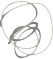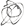Noppanit

29 Oct 2015

# Cosine Similarity for Dummies

Have you ever wonder how recommender system works? Or How Spotify or Amazon recommends what songs you might like or what product you might like to buy. I do. In this post, I’m going to try to explain how the recommendation algorithm works. First, let’s create a perfect scenario. I like to create an ideal example, it’s easier to understand.

Let’s say you have a very simple data of movies that users like collected from your site and you would like to match those people together based on their interests. How would you do that? One of the most popular methods is Cosine Similarity. When I first saw the name I was so confused; why Cosine? I remember when I was a kid I remembered my teacher told me about trigonometry so why does it have to do with that?

Here’s the sample data.

User 1 likes these movies

['Superman', 'Walking Dead', 'CSI']


User 2 likes these movies

['Superman', 'Walking Dead', 'CSI']


Even without any algorithm we can say that two users like the same movies. But we want the algorithm to tell us that the two users are very similar. Before we get into the mathematical formula world. We have to understand what a vector is?

# What’s a vector?

In Pyhsics, a vector has two things; magnitude and direction which can be written asI’d like to explain what a vector is but this site explains a lot better.

However, in Computer Science, 1-dimentional array is called a vector. But list in Python cannot perform vector operation so we have to use numpy or you have to build your own which I don’t recommend.

Now we know what a vector is but how does it relate to Cosine Similarity. In a nutshell, Cosine Similarity is a measure that calculates the cosine of the angle between them.

# Cosine SimilarityIn order to find the angle between the two vectors, we need to find the dot product of the two vectors as the formula below.

\begin{align} \text{cosine-similarity}(A,B) = \frac{\left<A,B\right>}{||A||\cdot||B||} \end{align}

# Show me the code

Ok. enough about explanation, show me the code.

import numpy as np

def cosin_sim(v, w):
return np.dot(v, w) / np.math.sqrt(np.dot(v, v) * np.dot(w, w))

# 1 if movie is in the list of movies and 0 is not.
cosin_sim([1, 1, 1], [1, 1, 1])
# 1.0



In the perfect example, we can see that the two users have the same interests.

Til next time,
noppanit at 21:35